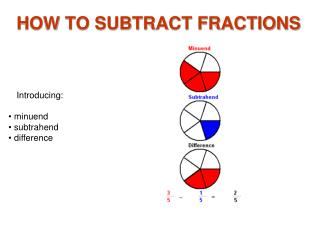DownloadDownload PresentationHOW TO SUBTRACT FRACTIONS

# HOW TO SUBTRACT FRACTIONS

Download Presentation## HOW TO SUBTRACT FRACTIONS

- - - - - - - - - - - - - - - - - - - - - - - - - - - E N D - - - - - - - - - - - - - - - - - - - - - - - - - - -
##### Presentation Transcript

1. HOW TO SUBTRACT FRACTIONS • Introducing: • minuend • subtrahend • difference

2. Subtract Fractions 1 This picture shows the minuend, subtrahend, and difference. The difference is what remains when the subtrahend is removed from the minuend.

3. Subtract Fractions 2 If the minuend and subtrahend have the same denominator, subtract the numerator of the subtrahend from the numerator of the minuend to get the numerator of the difference.

4. Subtract Fractions 3 The whole number 1 in 1 2/8 is subtracted from the whole number 2 in 2 5/8 for a whole number 1 in the difference. The fractions 5/8 and 2/8 are subtracted for 3/8 in the difference.

5. Subtract Fractions 4 Here the the subtrahend numerator in 7/8 is larger than the minuend numerator in 1 5/8. To subtract, the minuend is renamed as 13/8. Now the numerators in 13/8 and 7/8 can be subtracted. The difference6/8 is renamed in lowest terms as 3/4.

6. Subtract Fractions 5 The minuend 3 is renamed as 23/3 so that the numerators can be subtracted. This is done by decreasing the whole number 3 to 2 and renaming the 1 as 3/3. 3 = 2+1 = 2 + 3/3 .

7. Subtract Fractions 6 Here, unlike fractions are renamed as like fractions The minuend 3 1/4 is renamed as 3 2/8. Then the whole numbers and numerators are subtracted for a difference of 1 1/8.

8. Subtract Fractions 7 3 1/4 is renamed as 3 2/8 to make like fractions. The minuend 3 2/8 is then renamed as 2 10/8 so that the numerators can be subtracted. 3 2/8 = 2 + 1 + 2/8 = 2 + 8/8 + 2/8 = 2 10/8

9. Subtract Fractions 8 The same example with number lines shows that the minuend 3 1/4 is 5/8 larger than the subtrahend 2 5/8.

10. Subtract Fractions 9 Decreasing the subtrahend to 1 1/8 increased the difference to 2 1/8 . The smaller the subtrahend the larger the difference.

11. Subtract Fractions 10 What is the difference of 3 1/4 and 2 2/3 ?

12. Subtract Fractions 11 The difference of 3 1/4 and 2 2/3 is the distance from the end of the subtrahend to the end of the minuend . To find the difference from the image go straight down from the minuend number line onto the difference number line. The image shows how the difference is calculated